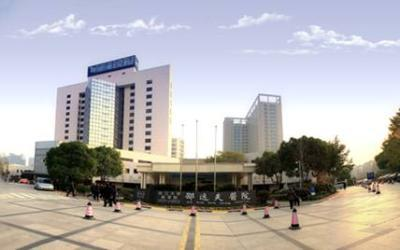# 医院主页• 消化内科
• 骨科
• 心内科
• 泌尿外科
• 普外科
• 神经内科
• 内分泌科
• 肿瘤内科
• 普外肝胆胃肠
• 耳鼻咽喉头颈外科
• 普外肝胆胰
• 普外科(下沙院区)
• 妇科
• 泌尿外科(下沙院区)
• 呼吸内科
• 妇产科
• 心内科(下沙院区)
• 肿瘤外科
• 肿瘤内科(下沙院区)
• 全科医学
• 神经外科
• 耳鼻咽喉科
• 眼科1
• 神经外科(下沙院区)
• 骨科(下沙院区)
• 肛肠外科
• 皮肤科
• 神经内科(下沙院区)
• 眼科
• 牙科
• 全科医学(下沙院区)
• 血液内科
• 肿瘤外科乳腺疾病
• 心胸外科(下沙院区)
• 肝病感染内科
• 消化内科(下沙院区)
• 肿瘤/乳腺外科（下沙院区
• 耳鼻咽喉科(下沙院区)
• 普外二科
• 种植牙门诊
• 胸外科
• 不孕不育门诊
• 疼痛门诊
• 乳腺甲状腺肿瘤外科
• 呼吸内科(下沙院区)
• 心胸外科
• 内分泌科(下沙院区)
• 心脏外科
• 乳腺甲状腺肿瘤(下沙院区)
• 肛肠外科(下沙院区)
• 肾内科
• 血液内科(下沙院区)
• 淋巴瘤门诊
• 皮肤科/痤疮门诊
• 肺癌及气管镜介入门诊
• 不孕不育中心
• 皮肤风湿病门诊(下沙院区)
• 关节与关节镜外科
• 皮肤过敏性疾病门诊
• 妇科(下沙院区)
• 心电图室
• 鼻功能障碍门诊
• 放射科
• 皮肤光动力治疗门诊
• 肿瘤放疗科
• 白癜风专科门诊
• 头颈外科
• 神经内科/睡眠门诊
• 乳腺/肿瘤外科
• 慢性萎缩性胃炎门诊
• 神经内科/帕金森病
• 牙周病门诊
• 精神卫生科
• 皮肤风湿病门诊
• 嗓音医学门诊
• 鼾症疾病门诊
• 介入治疗(下沙院区)
• 神经外科/垂体瘤门诊
• 普内科
• 血管外科
• 注射美容专科门诊
• 超声科
• 男性疾病门诊
• 骨质疏松门诊
• 呼吸/肺血管疾病门诊
• 肿瘤外科(下沙院区)
• 肾内科(下沙院区)
• 疼痛门诊(下沙院区)
• 炎症性肠病门诊
• 低颅压介入治疗
• 内分泌/垂体肾上腺性腺门诊
• 帕金森病术后调控
• 泌尿外科/结石
• 内分泌科妊娠糖尿病门
• 普外肝胆胰胃肠
• 呼吸/肺动脉高压
• 神经免疫病门诊
• 介入治疗
• 肿瘤内科淋巴瘤门诊XS
• 脱发门诊
• 耳聋门诊
• 耳科
• 消化道肿瘤筛查门诊
• 手足外科
• 房颤门诊
• 疼痛专科
• 鼻-鼻窦炎门诊
• 生殖医学中心
• 心血管内科
• 银屑病门诊
• 神经内科/记忆障碍门诊
• 乳腺甲状腺肿瘤外科(下沙院区)
• 头痛门诊
• 神经病痛及肌病门诊
• 头颈颌面肿瘤
• 普外乳腺胃肝胆
• 眼科2
• 影像诊断
• 皮肤科(下沙院区)
• 胃肠癌前疾病门诊
• 胸主动脉瘤外科
• 胰岛素泵治疗门诊
• 麻醉科
• 骨科下腰痛门诊
• 骨质疏松性骨折
• 血管外科(下沙院区)
• 整形外科
• 胎儿心超
• 静脉治疗护理
• 风湿免疫门诊
• 康复医学科(下沙院区)
• 康复医学科
• 中医科
• 精神卫生科(下沙院区)
• 核素治疗(下沙院区)
• 肿瘤放疗科(下沙院区)
• 不孕不育(男性)
• 眼科中心(下沙院区)
• 核素治疗门诊
• 甲状腺核素诊治门诊
• 核医学科
• 营养咨询门诊
• 失眠门诊
• 造口及疑难伤口咨询
• 风湿免疫(下沙院区)
• 疝病外科门诊
• 乳房整形门诊
• 肿瘤营养联合(下沙院区)
• 抗凝咨询门诊
• 微创涎腺外科门诊
• 足病门诊
• 卒中门诊
• 青光眼门诊
• 血透血管通路专病门诊
• 宫颈疾病门诊
• 腹膜透析专病(下沙院区)
• 血浆净化门诊
• 针灸门诊
• 泌尿系结石门诊
• 眩晕专科门诊
• 头颈肿瘤专科门诊
• 记忆门诊
• 妊娠合并内分泌疾病XS
• 牙科(下沙院区)
• 足下垂康复门诊
• 困难牙拔除门诊
• 皮肤性病门诊
• 鼻内镜术后复查门诊
• 冠心病术后随访门诊
• 中耳炎听力重建门诊
• 运动神经元病门诊
• 肉毒毒素治疗门诊
• 中医妇科
• 甲状腺门诊
• 面部年轻化门诊
• 心理咨询与治疗
• 心律失常门诊
• 乳腺疾病门诊
• 肝脏肿瘤联合门诊
• 换药(预约)
• 中医肿瘤
• 高血压门诊
• 正畸与牙颌发育门诊
• 肾病营养门诊(下沙院区)
• 妇科腔镜诊治门诊
• 肿瘤营养联合门诊
• 房颤术后随访门诊
• 慢性咳嗽/哮喘门诊
• 嗓音吞咽专科
• 营养咨询(下沙院区)
• 普外疝病门诊
• 眼眶-整形门诊
• 偏瘫、截瘫康复门诊
• 戒烟联合门诊
• 不孕不育/习惯性流产专科(下沙院区)
• 涎腺疾病专科门诊
• 医疗整形美容门诊
• 皮肤激光门诊
• 冠心病门诊
• 肝病感染内科(下沙院区)
• 前列腺增生筛查门诊
• 母乳喂养咨询门诊(下沙院区)
• 疤痕整形门诊